## Introduction

The high strength of graphene combined with its exceptional electronic1,2, optical3 and thermal properties4 has made it an ideal material for many fascinating applications, including flexible electronic displays5, corrosion-resistant coatings6, biological devices7,8 and many more9. While each of these applications exploits a different key property of graphene, they all implicitly depend on its exceptional mechanical properties for structural reliability10. However, such mechanical reliability of graphene is impacted by atomic defects in its structure. While the effect of relatively simple defects, such as isolated dislocation cores or a few special grain boundaries (GB), on the strength of graphene is understood11,12,13,14,15,16,17, the statistical fluctuations in strength and toughness due to the randomness in polycrystalline nanostructure remains largely unexplored.

Strength and toughness are arguably the two most important properties of a structural material. While strength is generally a function of the material’s resistance to deformation, toughness represents its resistance to fracture. In most materials, these properties tend to be mutually exclusive18. There are conflicting experimental reports whether the strength of polycrystalline graphene is actually a function of grain size19,20,21 making the role of simulation and theory more critical. Understanding these statistical fluctuations has become important in light of the fact that graphene synthesized with chemical vapour deposition (CVD) is polycrystalline, and this method is being used to manufacture more than 300,000 m2 of graphene annually22,23.

It is well-established that the strength and toughness of polycrystalline solids is strongly influenced by their granular structure. For instance, nanocrystalline metals are invariably significantly harder and much less ductile than their microcrystalline counterparts. This is due to the well-known ‘Hall–Petch effect’, wherein the motion of dislocations, and thereby plastic flow, is impeded by the presence of GB24. On the other hand, dislocations are typically not mobile in brittle materials, such as ceramics (and graphene), and thus the Hall–Petch effect is not observed in these materials. An entirely different mechanism leads to grain-size-dependent strength in brittle ceramics where the length of the typical extrinsic crack-like flaw (inclusion/porosity) relative to the grain size determines the characteristic strength25,26. In contrast to typical bulk brittle materials such as ceramics, graphene can be fabricated in a much cleaner and controlled environment, thus making the presence of large extrinsic defects unlikely22. In the absence of such extrinsic defects, the fluctuations in strength must arise from intrinsic atomic defects inherent to the granular nanostructure. The traditional theories developed for brittle ceramics with large extrinsic flaws are thus not applicable for strength fluctuations due to these intrinsic defects. In graphene these defects are GBs and triple junctions (TJs). Although a GB is an interface between crystalline regions of different lattice orientations and a TJ is the intersection of three such interfaces, in graphene GBs and TJs are typically composed of pentagon–heptagon defects, also known as five to seven defects (Fig. 1)27,28,29,30. These defects involve significant residual stresses and act like stress concentrators.

In this work, we develop an understanding of the grain-size-dependent statistical fluctuations in strength and toughness of polycrystalline graphene by using a combination of weakest-link statistics, continuum elastic theory and large-scale molecular dynamics (MD) simulations. Here we find that while the strength of a brittle polycrystalline graphene sheet is dictated by the weakest flaw in the entire sheet, its toughness is conversely influenced by the GB nearest to the crack tip. We develop theories to capture these two markedly different mechanisms. We believe that the theoretical framework developed here will be applicable to a large class of emerging two-dimensional (2D) materials.

## Results

### Statistical distribution of strength

We use MD simulations to gain insights into the statistical distribution of polycrystalline strengths31,32,33. We simulate fracture in over 19,000 polycrystalline graphene sheets with random grain shapes and orientations at several different combinations of sheet size, grain size and strain rate. The details of our simulations can be found in the Methods section and in Supplementary Note 1. Figure 2a shows a schematic representation of one such simulation. Figure 2b,c shows the snapshots of a polycrystalline simulation at zero stress, and at peak stress just before global failure, respectively. Residual stress at GB and TJ defects can be seen in Fig. 2b; the length-scale associated with these stresses is sub-nanometer, showing that the fluctuations are truly atomic-scale phenomena. Figure 2c shows that fracture originates at a grain boundary and then progresses through rest of the polycrystal. This observation is generic and fracture always originates at a GB or TJ in the several thousand polycrystals that we have simulated. This is not surprising since the interiors of the grains are defect-free and have no residual stresses. Once fracture originates, the incipient crack can extend in an intragranular or intergranular manner depending on the details of the grain orientation and loading direction.

Traditionally, the statistical distribution of fracture strengths in brittle materials is understood in terms of Weibull theory34,35,36,37. According to Weibull, the survival probability of brittle materials, defined as the probability that a volume V of the material survives at a stress σ, is of the form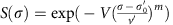where the lower-bound strength,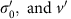are, respectively, the location and scale parameters, and m is the Weibull modulus. These parameters are generally evaluated by data fitting; however, in our case, fitting this from simulation data for each combination of grain size, strain rate and system size would give a different value for these parameters, and thus would not provide any physical insight into the dependence of these parameters on the nanostructural features such as grain size. As our goal is to gain such insight, we combine Kramer’s rate theory with extreme value statistics to derive an expression for the survival probability that explicitly accounts for the dependence on the physical atomistic and structural variables of interest. Similar expressions have been derived in various contexts previously, dating back at least to the work of Tobolsky and Eyring38, among other more recent studies39,40,41,42,43.

The specific details of our derivation can be found in the Supplementary Note 2; we present here the main result. We consider a polycrystalline graphene sheet of linear size L with a linear grain size μ. The sheet is loaded uniaxially at a constant strain rateat a fixed temperature of 300 K. Thus, the stress at time t is given by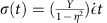, where Y is the Young’s modulus and η is the Poisson’s ratio. Our main result for polycrystalline strength gives the following expression for the survival probability of the sheet loaded at strain rate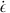up to a stress σ: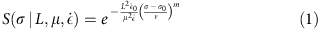where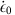is a reference strain rate for normalization, and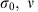are the rescaled location and scale parameters. As opposed to the usual Weibull form, in equation (1) the effect of structural parameters such as system size, grain size and loading rate is captured by a single non-dimensional parameter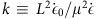, while the rescaled parameters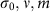are not affected by these details and are true material properties. We arrive at this particularly simple result by assuming that the individual defects in the graphene sheet are non-interacting, and that the loading is slow enough so that a thermal quasi-equilibrium is achieved. The dependence onis due to the fact that fracture initiates at GB and TJ defects, and the number of such defects in a graphene sheet scales as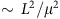. The factor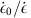is due to the fact that a lower strain rate gives more opportunity for thermal nucleation of fracture at the defects. It is interesting to note that similar ideas are applicable to dislocation nucleation in plasticity41,42,43,44,45,46, where it has been noted that the likelihood of survival of a metallic nanopillar under stress decreases with time due to nucleation events. Our model is a ‘weakest-link’ model, since we assume that the graphene sheet fractures as soon as its weakest GB/TJ defect becomes unstable. One particularly interesting outcome of our analysis is that the strength of graphene is a function of the ratio; thus there is no one well-defined value of strength at a given grain size. As we shall show, this behaviour is in contrast to the toughness, which is well-defined for a given grain size. Finally, it is worth noting that in our derivation, the connection between nucleation theory and Weibull analysis is made via the mathematical theory of extreme value statistics (as shown in Supplementary Equation 8)47,48.

We test our theoretical result with large-scale MD simulations. We perform extensive statistical sampling for 24 different combinations of the parameters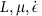. Figure 3a shows the variation of the survival probability with grain size, while Fig. 3b shows the effect of strain rate. A joint fit of the survival probability for the entire data set using the form given in equation (1) is obtained from the maximum likelihood estimator (see Supplementary Note 3 for details). This fit yields a Weibull modulus of m=10.7, a location parameter of σ0=19.5 GPa and a scale parameter of v=53.2 GPa. Note that the Weibull modulus and the scale parameter are a measure of the statistical variability of the strength, and the s.d. of the strength distribution scales as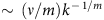. A modulus of 10 implies a moderate amount of variability from a structural engineering viewpoint. By comparison, the Weibull modulus for brittle glasses is 1–3, while that for well-designed ceramics is 10–20. The Weibull modulus has been interpreted in a similar manner in nanoscale plasticity, where it is considered to be a general measure of strength fluctuations44,46. The location parameter simply indicates a lower bound strength. Figure 3c shows that with the values of these parameters for graphene, all the data for the various combinations ofcollapses on single line, validating our theoretical result. Equation (1) further predicts that the mean fracture strength of graphene should scale as: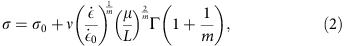where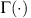is the Gamma function. Figure 3d presents a validation of this relation. Note that afterhave been obtained by fitting the survival probability to equation (1), there are no more free parameters, and thus the graph in Fig. 3d contains no free parameters. The scaling of the mean strength with grain size in equation (2) is similar to the result reported by Sha et al.49; however, they did not model the system size and strain-rate dependence.

### Statistical distribution of toughness

The strength of a material quantifies its failure in response to a state of large homogeneous stress, although in practice the state of stress is rarely homogeneous; rather, some regions of the material experience much higher stress than others. This is often due to the presence of stress singularities associated with sharp cracks and corners. The fracture toughness quantifies such failure in response to the stress singularity due to the presence of a crack. Not surprisingly, the toughness is one of the most important mechanical properties of a material. For nominally brittle materials, the fracture toughness can be evaluated in terms of the critical stress-intensity factor,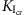, which is a measure of the strength of the stress singularity at the crack tip (the stress intensity specifically quantifies the magnitude of the elastic stress and displacement fields at the crack tip). Griffith’s criterion establishes a lower bound for the critical stress-intensity factor as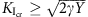, where γ is the energy required to create the fresh crack surface. However, the Griffith theory does not account for statistical fluctuations in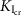due to variations in the local nanostructure of GBs and TJs. Here we develop a theory to account for these factors.

We use MD simulations to explore the statistical fluctuations in fracture toughness and its dependence on grain size in graphene. Figure 4a shows a schematic representation of our simulations. We simulate the initial advance of semi-infinite cracks in graphene polycrystals by imposing suitable fixed boundary conditions away from the crack tip, and evolving the atoms near the crack tip with canonical NVT dynamics; specific details can be found in the Methods section. As shown in Fig. 4b, the stress singularity at the crack tip interacts with the GB and TJ defects in the immediate vicinity of the tip. The critical stress-intensity factor needed to initiate crack advance depends on the local nanostructure; as an arbitrary crack would experience a random sampling of this nanostructure, there is significant statistical spread in the values ofand thus it is meaningful to define a grain-size-dependent survival probability,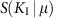, as the probability that a polycrystal with grain size μ survives an applied stress-intensity factor KI. Figure 5b shows the numerically obtained survival probability from our MD analysis on polycrystals with different grain sizes. This figure clearly shows that although there is significant spread in the observations, there is a strong dependence on grain size.

In general, we have observed that cracking progresses by breaking the bonds associated with the pentagon–heptagon defects near the crack tip. In fact, due to lack of plasticity, the stress concentration at the crack tip in graphene is so strong that the first bond to break is almost always within 10 Å of the crack tip. Because the polycrystalline morphology is generated randomly, as the grain size is increased it becomes more likely that there are no defects near the crack tip. If there are no GBs or TJs near the tip, then the toughness is simply given by the toughness of the monocrystal that contains the crack tip. Since the monocrystal will have an arbitrary orientation, a part of the statistical spread in our simulation results is due to the variation in fracture toughness of pristine graphene with orientation. For the AIREBO empirical potential50,51 used in our MD simulations, we find that the elastic modulus Y=858 GPa, and γ varies between 5.9 and 6.3 J m−2 for single crystals depending on the orientation, resulting in an orientation-dependent Griffith estimate of fracture toughness between 3.2 and 3.3 MPa m1/2 for monocrystalline graphene. In practice the observed values of the toughness of brittle solids are slightly higher than the Griffith bound due to such effects as lattice trapping and crack roughness. We simulate crack advance in graphene monocrystals with random orientations and find that the resulting distribution of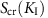is well-described by a Gaussian distribution with mean 3.9 MPa·m1/2 and s.d. 0.4 MPa·m1/2. This somewhat accounts for the behaviour in the upper tail (large KI) of Fig. 5b, but the interesting grain-size-dependent behaviour for smaller values of KI needs to be explored further. We note that the trend of increasing toughness with grain size is opposite to the trend reported elsewhere52. Presumably, this is due to the fact that we are measuring the value ofstrictly as a crack-initiation toughness (at instability) while ref. 52 reports a propagation toughness; moreover, their simulations seem to display crack bridging with single atom carbon chains (a non-physical phenomenon typical of the AIREBO potential, which we avoid in this work by the design of our simulations).

We now develop a theory to explain the grain-size dependence ofin graphene. To maintain theoretical tractability, we do not account for loading-rate dependence, and all our simulations of initial crack advance are performed in the quasi-static limit. The stress field in the region of K-dominance is known from linear-elastic fracture mechanics (LEFM). For ductile materials with brittle inclusions, the statistical variation in toughness can be obtained by integrating the Weibull-type expression of stress survival probability equation (1) over the LEFM crack-tip stress field53,54. A similar method has been used to study the nucleation of dislocations under an indenter tip in plasticity44,46. However, this technique implicitly assumes that a large population of defects is sampled by each crack tip (or indenter tip in plasticity); while this assumption is valid for ductile materials with large crack-tip process zones, it is clearly violated for brittle materials like graphene where the crack tip samples only a few defects within a few angstroms of the tip. Thus, a new approach is needed. It can be argued that the effect of TJs on the distribution of KI should be minimal, since the probability of finding a TJ near a crack tip is much smaller than the probability of finding a GB nearby. Figure 5a shows a schematic representation of our model. We consider an arbitrary GB a distance l ahead of the crack tip, inclined at an angle φ to the crack. Each point on this GB experiences a different normal stress σn that can be calculated from LEFM to be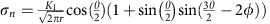. We define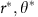as the point at which the GB experiences the maximum normal stress,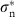. Note thatis implicitly a function of l since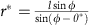. We make the assumption that this GB survives at stress intensity KI if it can survive the normal stress. We measure the GB survival probability,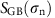, for applied normal stress by simulating over 4,000 GBs (see Methods, Supplementary Note 1 and Supplementary Fig. 1); the resulting survival probability and probability density are shown in Fig. 5c. The polycrystalline survival probability can then be written as: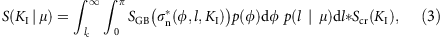whereis the probability density of the random GB orientation angle φ,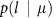is the grain-size-dependent probability density of the GB distance from crack tip, and lc is a lower cutoff due to the discreteness of the lattice. Equation (3) essentially means that the polycrystal survives a stress intensity KI if the nearest GB ahead of the crack tip and the crystal containing the crack survive. Since the polycrystal has random boundary orientations, the distribution of φ is uniform, giving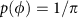. The distribution of the GB distance from the crack tip is measured from the randomly generated polycrystals to be a half-Gaussian distribution,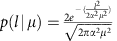, where the parameter α is equal to 0.64. A collapse of the probability density of the measured distance to the nearest GB ahead of the crack tip according to this form is shown in Fig. 5d. Finally, we take lc to be equal to the distance between the centres of the next nearest hexagons in graphene (=3a, where a is the carbon–carbon bond length in graphene). Note that there are no free parameters in equation (3). However, since it is unreasonable to assume that only the nearest GB directly ahead of the crack tip contributes to fracture, we leave α as a free parameter when fitting equation (3) to numerical data. The solid lines in Fig. 5b show a comparison of the predictions of the grain-size-dependent polycrystalline fracture toughness obtained from equation (3) with the numerical data obtained from extensive simulation. It should be noted that α=0.7 is obtained from the fitting process, and is very close to the measured value of 0.64. Thus, we are able to derive a formula for the statistical fluctuations in polycrystalline toughness that has only one free parameter, the value of which can also be measured to good accuracy from the polycrystal geometry.

## Discussion

Our theoretical results offer important insights into the grain size and sample-size dependence of strength and toughness of graphene. The scaling of strength with grain size in traditional metals is understood in terms of the Hall–Petch effect24, while the analogous results in ceramics are explained with the inverse square-root scaling25. The current paper provides the corresponding scaling relations for the strength of graphene and other brittle 2D materials equations (1) and (2), while equation (3) provides the scaling relation for the toughness of 2D materials. As such, these relationships can serve a role analogous to the Hall–Petch effect in design of 2D materials. An understanding of the scaling relations is needed to design any device where the structural integrity of a graphene component is to be ensured. Their role is actually more significant in 2D materials because it is difficult to conduct systematic experimental investigations of their mechanical properties. The fact that the strength of polycrystalline graphene has never been measured under uniaxial tension, and that its toughness has been measured only once, is a true testament to this experimental challenge.

Our prediction of a mean toughness of 3–4 MPa·m1/2 compares favourably with the only reported experimental measurement of the toughness of graphene10. Note that this toughness is not high; specifically, it is three to four times tougher than silicon and pyrolytic carbon55 yet 20 to 50% less tough than polycrystalline diamond56. We have found that the distribution of toughness, as well as strength, in polycrystalline graphene is strongly dependent on the grain size. However, at larger grain sizes the distribution of toughness becomes less sensitive to grain size. We predict that for grain sizes larger than 256 Å, the toughness will be essentially independent of the grain size. Thus the toughness dependence of grain size is a phenomena limited to nanocrystalline graphene. In contrast, the strength will continue to be grain-size and sample-size dependent, and the experimental results will have to be scaled with the Weibull from equation (1) to get true material properties. We predict a Weibull modulus of 10 for polycrystalline graphene. We note that even though our simulations are for nanocrystalline grains, we expect our results to be valid for much larger micrometer-sized grains, since no new physics is expected to emerge at the intermediate length scales. Our results are consistent with the observations that presence of GB reduces the strength of polycrystalline graphene20,21. Finally, we note that our simulations consider only the equilibrium geometrically necessary dislocations, and ignore the non-equilibrium defects such as disclinations57,58. It has been demonstrated that these latter defects can have a significant effect on the strength of graphene57,58. Our theory can be modified to include the effect of such defects if the statistical information regarding them, such as their density, can be obtained experimentally.

What do these results mean in practical terms? The first measurement of the strength of pristine monocrystalline graphene reported an intrinsic strength of about 130 GPa, and a Young’s modulus of about 1 TPa. In practical terms these results mean that a soccer ball can be placed on a single sheet of graphene without breaking it. What object can be supported by a corresponding sheet of polycrystalline graphene? It turns out that a soccer ball is much too heavy, and polycrystalline graphene can only support a ping-pong ball. Still remarkable for a one-atom-thick material, but not quite as breathtaking anymore.

## Methods

### Details of simulations

All numerical simulations were performed with the LAMMPS59 code by using the AIREBO50,51 empirical interatomic potential, with the interaction cutoff parameter set to 1.92 Å (ref. 11). The simulations were conducted in the NVT ensemble (the canonical ensemble with constant number of particles, volume and temperature) at temperature T=300 K. The random grain morphology was generated by randomly choosing the required number of grain ‘centres’ and generating the boundaries of the granular domains with a Voronoi construction. The crystalline orientations within each grain were also taken to be random. The atomic positions, particularly at the GB, were generated by a recently proposed algorithm that yields well-annealed GBs60. Even though this algorithm was proposed for generation of GBs, it can be used successfully to generate well-annealed polycrystalline samples (see Supplementary Note 1 and Supplementary Figs 2 and 3). All structures used in our strength and toughness simulations were prepared running NVT dynamics at T=300 K for 1 ps, followed by energy minimization using the conjugate gradient algorithm (allowing out of plane deformations, and allowing the simulation cell dimensions to change to attain zero stress).

### Simulation of strength

For the simulations of polycrystalline strength, a constant strain ratewas imposed using the SLLOD equations61 as implemented in LAMMPS, and the stress response was measured; the largest observed stress was taken to be the ultimate strength of the sample. We performed simulations for the following 24 combinations of the parameters: (64,32,1), (128,64,1),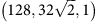,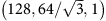, (128,32,1),,,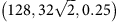,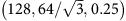, (256,128,1),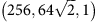, (256,64,1),,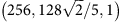, (256,32,1),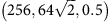,,, (256,32,0.5),,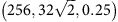,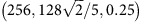, (256,32,0.25), (512,32,1), where the units of L,μ are Å, and those ofare 109 s−1. The number of simulations performed for statistical sampling was 104, 103, 102 for L=64,128,256,512 Å, respectively. See Supplementary Figs 4 and 5 for the stress–strain response and snapshots of a representative simulation.

### Simulation of toughness

For polycrystalline toughness, we simulated systems of length L=256 Å with a crack tip placed at the centre of the simulation box. The atoms outside a radius of 100 Å from the crack tip were fixed according to the LEFM K-field displacement solution,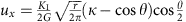,, uz=0, where G is the shear modulus and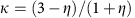. The atoms within a radius of 100 Å of the crack tip were evolved with NVT dynamics at temperature T=300 K. The radius of 100 Å is chosen because the applied strain beyond this radius is <0.02, which is small enough for linear elasticity to be applicable. Also, we note that all bond breaking events occur within a radius of 20 Å, thus the simulation box size is large enough to avoid any finite size effects. The applied stress-intensity factor KI was increased in increments of 0.1 MPa·m1/2. The system was held at each value of KI for t=1 ps. The critical stress–intensity factor was taken to be the lowest value of KI for which the crack grows. We simulated initial crack advance in polycrystals with grain size μ=16,32,64 Å. For each grain size, crack advance was simulated in 500 polycrystals with random grain morphology.